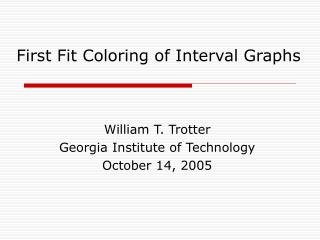# First Fit Coloring of Interval Graphs - PowerPoint PPT PresentationDownload PresentationFirst Fit Coloring of Interval Graphs

First Fit Coloring of Interval Graphs
Download Presentation## First Fit Coloring of Interval Graphs

- - - - - - - - - - - - - - - - - - - - - - - - - - - E N D - - - - - - - - - - - - - - - - - - - - - - - - - - -
##### Presentation Transcript

1. First Fit Coloring of Interval Graphs William T. Trotter Georgia Institute of Technology October 14, 2005

2. Interval Graphs

3. Interval Graphs are Perfect Χ = ω = 4

4. What Happens with Another Order?

5. On-Line Coloring of Interval Graphs Suppose the vertices of an interval graph are presented one at a time by a Graph Constructor. In turn, Graph Colorer must assign a legitimate color to the new vertex. Moves made by either player are irrevocable.

6. Optimal On-Line Coloring • Theorem (Kierstead and Trotter, 1982) • There is an on-line algorithm that will use at most 3k-2 colors on an interval graph G for which the maximum clique size is at most k. • This result is best possible. • The algorithm does not need to know the value of k in advance. • The algorithm is not First Fit. • First Fit does worse when k is large.

7. Dynamic Storage Allocation

8. How Well Does First Fit Do? • For each positive integer k, let FF(k) denote the largest integer t for which First Fit can be forced to use t colors on an interval graph G for which the maximum clique size is at most k. • Woodall (1976) FF(k) = O(k log k).

9. Upper Bounds on FF(k) Theorem: Kierstead (1988) FF(k) ≤ 40k

10. Upper Bounds on FF(k) Theorem: Kierstead and Qin (1996) FF(k) ≤ 26.2k

11. Upper Bounds on FF(k) Theorem: Pemmaraju, Raman and Varadarajan(2003) FF(k) ≤ 10k

12. Upper Bounds on FF(k) Theorem: Brightwell, Kierstead and Trotter (2003) FF(k) ≤ 8k

13. Upper Bounds on FF(k) Theorem: Narayansamy and Babu (2004) FF(k) ≤ 8k - 3

14. Analyzing First Fit Using Grids

16. Academic Algorithm - Rules • A Belongs to an interval • B Left neighbor is A • C Right neighbor is A • D Some terminal set of letters has more than 25% A’s • F All else fails.

17. A Pierced Interval A B C C D B A

18. The Piercing Lemma Lemma: Every interval J is pierced by a column of passing grades. Proof: We use a double induction. Suppose the interval J is at level j. We show that for every i = 1, 2, …, j, there is a column of grades passing at level i which is under interval J

19. Double Induction

20. Initial Segment Lemma Lemma: In any initial segment of n letters all of which are passing, a ≥ (n – b – c)/4

21. A Column Surviving at the End • b ≤ n/4 • c ≤ n/4 • n ≥ h+3 • h ≤ 8a - 3

22. Lower Bounds on FF(k) Theorem: Kierstead and Trotter (1982) There exists ε > 0 so that FF(k) ≥ (3 + ε)k when k is sufficiently large.

23. Lower Bounds on FF(k) Theorem: Chrobak and Slusarek (1988) FF(k) ≥ 4k - 9when k ≥ 4.

24. Lower Bounds on FF(k) Theorem: Chrobak and Slusarek (1990) FF(k) ≥ 4.4 k when k is sufficiently large.

25. Lower Bounds on FF(k) Theorem: Kierstead and Trotter (2004) FF(k) ≥ 4.99 k when k is sufficiently large.

26. A Likely Theorem Our proof that FF(k) ≥ 4.99 k is computer assisted. However, there is good reason to believe that we can actually write out a proof to show: For every ε > 0, FF(k) ≥ (5 – ε) k when k is sufficiently large.

27. Tree-Like Walls

28. A Negative Result and a Conjecture However, we have been able to show that the Tree-Like walls used by all authors to date in proving lower bounds will not give a performance ratio larger than 5. As a result it is natural to conjecture that As k tends to infinity, the ratio FF(k)/k tends to 5.